Next: Electron Diffraction Up: Wave Mechanics Previous: Introduction

# Photoelectric Effect

The so-called photoelectric effect, by which a polished metal surface emits electrons when illuminated by visible or ultra-violet light, was discovered by Heinrich Hertz in 1887. The following facts regarding this effect can be established via careful observation. First, a given surface only emits electrons when the frequency of the light with which it is illuminated exceeds a certain threshold value that is a property of the metal. Second, the current of photoelectrons, when it exists, is proportional to the intensity of the light falling on the surface. Third, the energy of the photoelectrons is independent of the light intensity, but varies linearly with the light frequency. These facts are inexplicable within the framework of classical physics.

In 1905, Albert Einstein proposed a radical new theory of light in order to account for the photoelectric effect. According to this theory, light of fixed angular frequency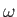consists of a collection of indivisible discrete packages, called quanta,C.1 whose energy is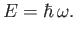(C.1)

Here,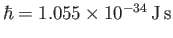is a new constant of nature, known as Planck's constant. (Strictly speaking, it is Planck's constant divided by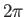). Incidentally,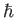is called Planck's constant, rather than Einstein's constant, because Max Planck first introduced the concept of the quantization of light, in 1900, when trying to account for the electromagnetic spectrum of a black body (i.e., a perfect emitter and absorber of electromagnetic radiation).

Suppose that the electrons at the surface of a piece of metal lie in a potential well of depth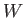. In other words, the electrons have to acquire an energyin order to be emitted from the surface. Here,is generally called the workfunction of the surface, and is a property of the metal. Suppose that an electron absorbs a single quantum of light, otherwise known as a photon. Its energy therefore increases by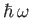. Ifis greater thanthen the electron is emitted from the surface with the residual kinetic energy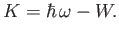(C.2)

Otherwise, the electron remains trapped in the potential well, and is not emitted. Here, we are assuming that the probability of an electron absorbing two or more photons is negligibly small compared to the probability of it absorbing a single photon (as is, indeed, the case for relatively low-intensity illumination). Incidentally, we can determine Planck's constant, as well as the workfunction of the metal, by plotting the kinetic energy of the emitted photoelectrons as a function of the wave frequency. This plot is a straight-line whose slope is, and whose intercept with theaxis is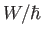. Finally, the number of emitted electrons increases with the intensity of the light because, the more intense the light, the larger the flux of photons onto the surface. Thus, Einstein's quantum theory of light is capable of accounting for all three of the previously mentioned observational facts regarding the photoelectric effect. In the following, we shall assume that the central component of Einstein's theory--namely, Equation (C.1)--is a general result that applies to all particles, not just photons.Next: Electron Diffraction Up: Wave Mechanics Previous: Introduction
Richard Fitzpatrick 2016-01-25